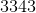# ISEE Middle Level Math : How to find mode

## Example Questions

← Previous 1 3 4 5 6 7 8 9 12 13

### Example Question #1 : How To Find Mode

On the last day of school,Adam asked each of his classmates how many days of school they missed for the entire school year.  He created a stem-and-leaf plot with the information.

0 | 0 0 2 4 5 6 8

1 | 0 0 1 2

2 | 3

4 | 7

What is the mode(s) of the stem-and-leaf plot?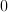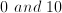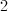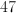Explanation:

The mode of a set of data is the value that occurs the most frequently.

Here, 0 occurs twice on the first line, and twice on the second line.  These are different values since the left side of the vertical line represents the ten's place.  So on the first line, the "0" represents 0, and on the second line the "0" represents 10.  So 0 occurs twice, and ten occurs twice.

It is possible to have more than one mode.  If multiple values occur more than once, occur the same number of times as each other, and occur more than any other values, then they are both modes.

### Example Question #433 : Isee Middle Level (Grades 7 8) Mathematics Achievement

What is the mode of 43, 23, 56, 44, 47, and 43?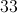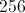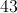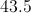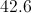Explanation:

The mode is the number that appears most frequently.  All the numbers appear once, except for 43, which occurs twice.  43 is the mode of this number set.

### Example Question #434 : Isee Middle Level (Grades 7 8) Mathematics Achievement

Find the mode: 45, 38, 29, 12, 38, 20, 13.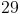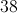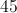Explanation:

Identlfy the number that appears most frequently in the set.

### Example Question #435 : Isee Middle Level (Grades 7 8) Mathematics Achievement

Find the mode: 213, 144, 213, 788, 532, 532, 965, 690, 532, 714.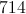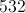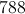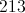Explanation:

Identlfy the number that appears most frequently in the set.

The answer is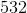.

### Example Question #436 : Isee Middle Level (Grades 7 8) Mathematics Achievement

Find the mode in this set of numbers:

7435, 8976, 2784, 7435, 1289, 8976, 2110, 8992, 8976, 2784.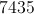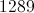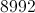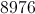Explanation:

Find the most recurring number in the set.

The answer is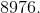### Example Question #437 : Isee Middle Level (Grades 7 8) Mathematics Achievement

Find the mode in this set of numbers:

22, 24, 28, 23, 27, 24, 29, 35, 23, 42, 42, 24, 26, 25, 26.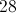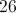Explanation:

Find the number that appears most frequently.

### Example Question #1 : How To Find Mode

Fidn the mode in this set of numbers:

112, 121, 123, 132, 121, 211, 120, 122, 121, 131, 133, 113, 311, 121, 211.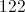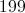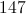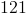Explanation:
 Find the number that appears most frequently.

### Example Question #439 : Isee Middle Level (Grades 7 8) Mathematics Achievement

Find the mode in this set of numbers:

9881, 9889, 9818, 9981, 9891, 9918, 9989, 9889, 9198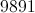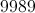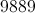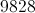Explanation:

Identify the most recurring number in the set: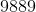### Example Question #440 : Isee Middle Level (Grades 7 8) Mathematics Achievement

Find the mode in this set of numbers:

2521, 2512, 2525, 2552, 2551, 2525, 2515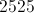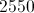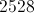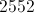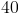Explanation:

First, order the numbers from least to greatest: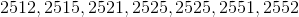Identify the most recurring number: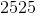### Example Question #1 : How To Find Mode

Find the mode in this set of numbers:

3343, 4434, 4334, 3343, 4343, 3434, 3334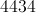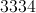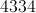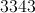Identify the most recurring number: